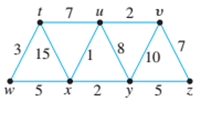Chapter 10.6, Problem 10ES### Discrete Mathematics With Applicat...

5th Edition
EPP + 1 other
ISBN: 9781337694193

#### Solutions

Chapter
Section### Discrete Mathematics With Applicat...

5th Edition
EPP + 1 other
ISBN: 9781337694193
Textbook Problem
1 views

# For each of the graphs in 9 and 10, find all minimum spanning trees that can be obtained using (a) Kruskal’s algorithm and (b) Prim’s algorithm starting with vertex a or t. Indicate the order in which edges are added to form each tree. 10.To determine

(a)

For the graph, find all minimum spanning trees that can be obtained using

Kruskal’s algorithm. Indicate the order in which edges are added to form each tree.

Explanation

Given info:

Calculation:

Kruskal’s algorithm:

• Start from a graph T that contains only the vertices and no edges.
• Repeatedly select the edge in the graph G with the smallest weight (that doesn’t cause a circuit) and add it to the graph T.
• Once the graph is connected. We have found a minimum spanning tree. We ignore all edges that were previously added to the graph.

First iteration: The edge with the smallest weight is the edge between u and x with weight 1, thus we add the edge [u,x] to the minimum spanning tree.

Second iteration: the edges with the smallest weight are the edges between x and y and the edge between u and v with weight 2 each. 2 will choose to add the edge [u,v] first to the minimum spanning tree. Note: You could also choose to use the edge [x,y] first.

Third iteration: The edge with the smallest weight is the edge between x and y with weight 2, thus we add the edge [a,e] to the minimum spanning tree.

Forth iteration: The edge with the smallest weight is the edge between t and w with weight 3, thus we add edge [t,w] to the minimum spanning tree.

Fifth iteration: The edges with the smallest weight are the edge between w and x and the edge between y and z with weight 5 each. I will choose to add the edge [w,x] to the minimum spanning tree. Note: You could also choose to use the edge [c,e] instead.

Sixth iteration: The edge with the smallest weight is the edge between y and z with weight 5, thus we add the edge [y,z] to the minimum spanning tree

To determine

(b)

Find all minimum spanning trees that can be obtained using Prim’s algorithm starting with vertex a or t. indicate the order in which edges are added to form each tree.

### Still sussing out bartleby?

Check out a sample textbook solution.

See a sample solution

#### The Solution to Your Study Problems

Bartleby provides explanations to thousands of textbook problems written by our experts, many with advanced degrees!

Get Started